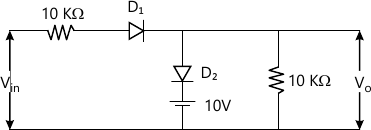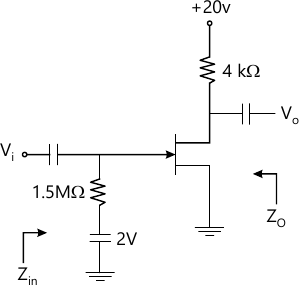MORE IN Analog Electronics
Total marks: --
Total time: --
INSTRUCTIONS
(1) Assume appropriate data and state your reasons
(2) Marks are given to the right of every question
(3) Draw neat diagrams wherever necessary

1 (a) With necessary equivalent circuit, explain the various diode equivalent circuits.
6 M
1 (b) What do you understand by reverse recovery time? Explain its importance in selection of diode for an application.
6 M
1 (c) For the diode circuit shown in Fig. Q1(c) draw the transfer characteristics. The input is 40 sin ωt. Show clearly the steps of analysis. All diodes are ideal.8 M

2 (a) Discuss the effect of varying IB and VCC on the Q-point. Explain your answer with relevant diagram.
6 M
2 (b) An emitter bias circuit has RC=2kΩ, RE=680 Ω, VE=2.1 V, VCE=7.3 V IB=20 μA. Find VCC, RB and β.
6 M
2 (c) A voltage divider biased circuit has R1=39 kΩ, R2=8.2 KΩ, RC=3.3 KΩ, RE=1 kΩ, VCC=18V. The silicon transistor used has β=120. Find Q-point and stability factor.
8 M

3 (a) Derive an expression for voltage gain, input impedance and output impedance of an emitter follower amplifier using re-model.
6 M
3 (b) A voltage divider biased amplifier has R1=82 kΩ, R2=22 kΩ, RE=1 kΩ, RC=2.2 KΩ. VCC=18 V. The silicon transistor β=100. Take RS=1 kΩ, RL=5.6 kΩ. Find voltage gain, input impedance, output impedance.
6 M
3 (c) A transistor in CE mode has hie=1100 Ω, hfe=100, hre=2.5×10-4, hoe=25 μ℧. Find voltage gain, input impedance and output impedance. Take RS=1 kΩ, RL=1 kΩ. Also find current gain.
8 M

4 (a) Discuss with relevant equivalent circuit the method of determination of lower cutoff frequency for a voltage divider biased CE amplifier.
10 M
4 (b) A voltage divider biased CE amplifier has RS=1 kΩ, R1= 40 kΩ, R2=10 kΩ, RE=2 kΩ, RC=2.2 kΩ, CS=10 μF, CC=1 μF, CE=20 μF, β=100, VCC=20. The parasitic capacitance are Cπ(Cbe)=36 pF, Cμ(Cbe)=4 pF, Cce=1 pF, Cwi=6 pF, Cwo=8 pF. Determine higher cutoff frequency.
10 M

5 (a) Obtain expression for voltage gain, input impedance and output impedance of a Darlington emitter follower. Draw necessary equivalent circuit.
8 M
5 (b) Mention the different configuration of feedback amplifiers and obtain expression for voltage gain with feedback for any one configuration.
6 M
5 (c) What are the advantages of cascading amplifiers? Obtain expression for overall voltage gain for an n-stage cascaded amplifier.
6 M

6 (a) Prove that the maximum conversion efficiency of class A transformer coupled amplifier is 50%.
8 M
6 (b) With neat diagram, explain the methods of obtaining phase shift of input signal for class B operation.
6 M
6 (c) The harmonic distortion component in an power amplifier is D2=0.1, D3=0.02, D4=0.03. The fundamental current amplitude is 4 A and it supplies a load of 8Ω. Find total harmonic distortion, fundamental power and total power.
6 M

7 (a) What is Barkhausen for sustained oscillator? Explain basic principle of operation of oscillators.
8 M
7 (b) With a neat circuit diagram, explain the working of Hartley oscillator. Write the equation for frequency of oscillations.
8 M
7 (c) A crystal has mounting capacitance of 10 pF. The inductance equivalent of mass is 1 mH, the frictional resistance = 1 kΩ and compliance = 1pF. Find series and parallel resonant frequency.
4 M

8 (a) Obtain the expression for voltage gain, input impedance output impedance for a JfET common source amplifier with self-bias configuration.
8 M
8 (b) For the FET amplifier in Figure, find voltage gain, input impedance and output impedance. The FET has IDSS=15 mA, Vp=-6V, YOS=25 μS.8 M
8 (c) Mention the difference between BJT and FET.
4 M

More question papers from Analog Electronics